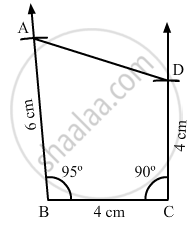Share

# Construct a Quadrilateral Abcd, in Which Ab = 6 Cm, Bc = 4 Cm, Cd = 4 Cm, ∠B = 95° and ∠C = 90°. - Mathematics

Course

#### Question

Construct a quadrilateral ABCD, in which AB = 6 cm, BC = 4 cm, CD = 4 cm, ∠B = 95° and ∠C = 90°.

#### Solution

Steps of construction:

Step I: Draw BC = 4 cm .

Step II: Construct ∠ABC = 95° at B .

Step III : With B as the centre and radius 6 cm, cut off BA = 6 cm .

Step IV: Construct ∠BCD = 90° at C .

Step V : With C as the centre and radius 4 cm, cut off BA = 4 cm .

Step VI: Join CD .